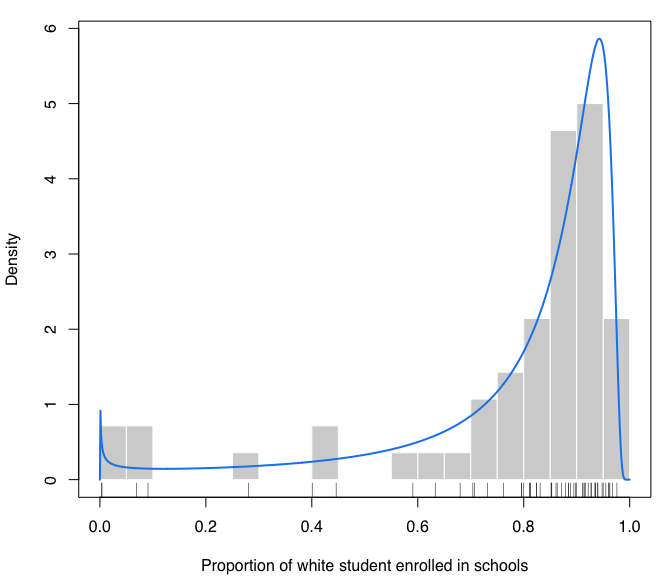# Introduction

mclustAddons is a contributed R package that extends the functionality available in the mclust package (Scrucca et al. 2016).
In particular, the following methods are included:

• density estimation for data with bounded support (Scrucca, 2019);

• modal clustering using modal EM algorithm for Gaussian mixtures (Scrucca, 2021);

• entropy estimation via Gaussian mixture modeling (Robin & Scrucca, 2023).

This document gives a quick tour of mclustAddons (version 0.7.2). It was written in R Markdown, using the knitr package for production.

References on the methodologies implemented are provided at the end of this document.

``````library(mclustAddons)
## Package 'mclust' version 6.0.0
## Type 'citation("mclust")' for citing this R package in publications.

# Density estimation for data with bounded support

## Univariate case with lower bound

``````x <- rchisq(200, 3)
xgrid <- seq(-2, max(x), length=1000)
f <- dchisq(xgrid, 3)  # true density
dens <- densityMclustBounded(x, lbound = 0)
summary(dens, parameters = TRUE)
## ── Density estimation for bounded data via GMMs ───────────
##
## Boundaries:   x
##       lower   0
##       upper Inf
##
## Model E (univariate, equal variance) model with 1 component
## on the transformation scale:
##
##  log-likelihood   n df       BIC       ICL
##       -390.0517 200  3 -795.9983 -795.9983
##
##                                     x
## Range-power transformation: 0.3715163
##
## Mixing probabilities:
## 1
## 1
##
## Means:
##         1
## 0.9191207
##
## Variances:
##        1
## 1.309037
plot(dens, what = "density")
lines(xgrid, f, lty = 2)``````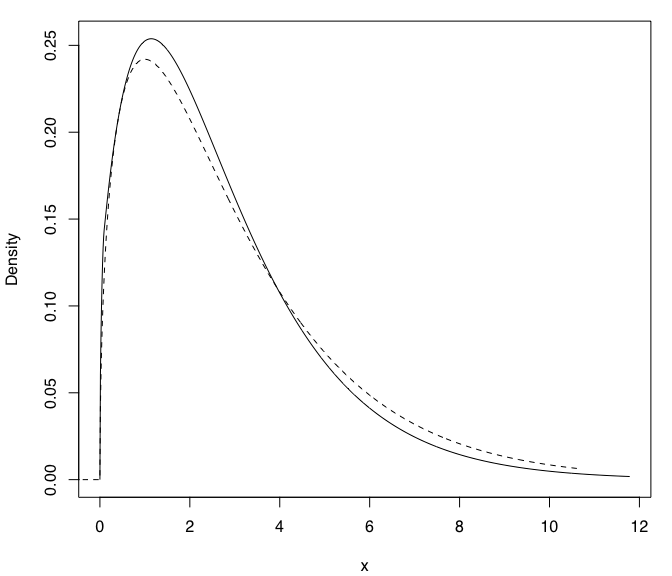``plot(dens, what = "density", data = x, breaks = 15)``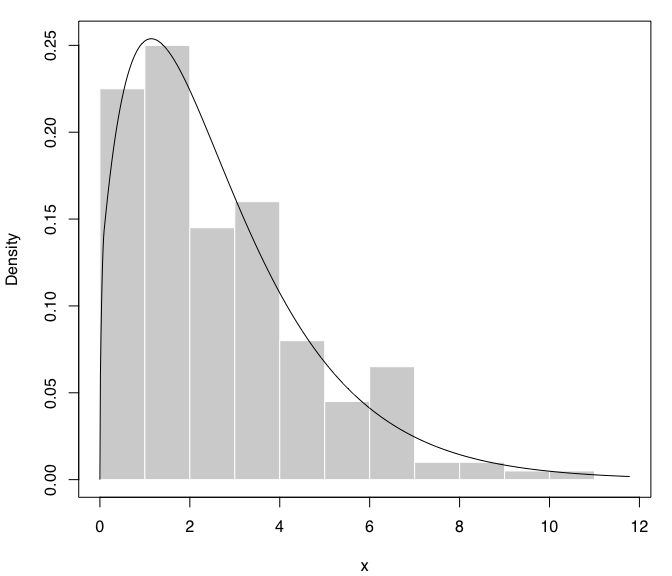## Univariate case with lower & upper bounds

``````x <- rbeta(200, 5, 1.5)
xgrid <- seq(-0.1, 1.1, length=1000)
f <- dbeta(xgrid, 5, 1.5)  # true density
dens <- densityMclustBounded(x, lbound = 0, ubound = 1)
summary(dens, parameters = TRUE)
## ── Density estimation for bounded data via GMMs ───────────
##
## Boundaries: x
##       lower 0
##       upper 1
##
## Model E (univariate, equal variance) model with 1 component
## on the transformation scale:
##
##  log-likelihood   n df      BIC      ICL
##        104.4678 200  3 193.0407 193.0407
##
##                                      x
## Range-power transformation: -0.1600959
##
## Mixing probabilities:
## 1
## 1
##
## Means:
##        1
## 1.164398
##
## Variances:
##         1
## 0.7221851
plot(dens, what = "density")``````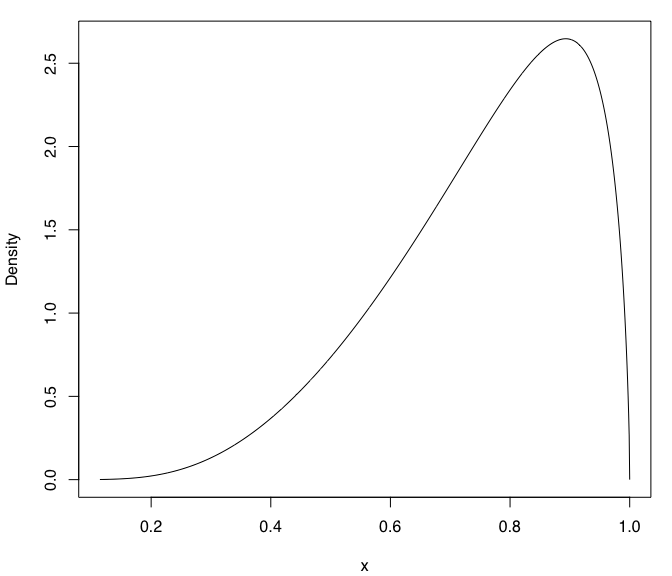``plot(dens, what = "density", data = x, breaks = 11)``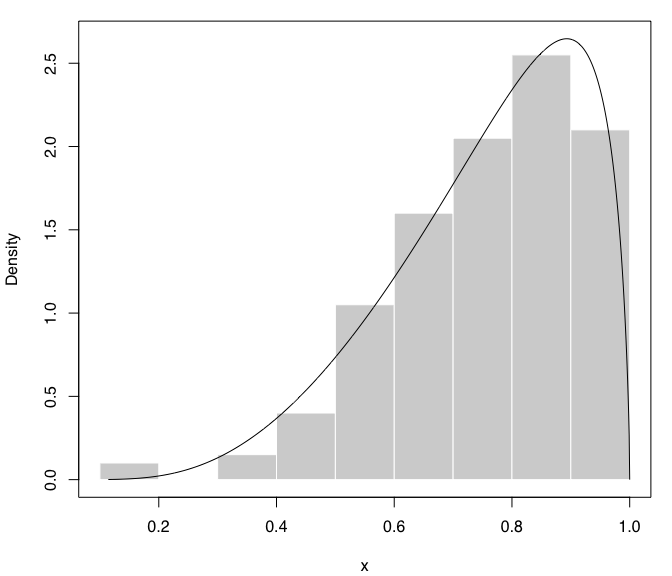## Bivariate case with lower bounds

``````x1 <- rchisq(200, 3)
x2 <- 0.5*x1 + sqrt(1-0.5^2)*rchisq(200, 5)
x <- cbind(x1, x2)
dens <- densityMclustBounded(x, lbound = c(0,0))
summary(dens, parameters = TRUE)
## ── Density estimation for bounded data via GMMs ───────────
##
## Boundaries:  x1  x2
##       lower   0   0
##       upper Inf Inf
##
## Model VEE (ellipsoidal, equal shape and orientation) model with 1 component
## on the transformation scale:
##
##  log-likelihood   n df       BIC       ICL
##       -882.0264 200  7 -1801.141 -1801.141
##
##                                    x1        x2
## Range-power transformation: 0.2835466 0.3094675
##
## Mixing probabilities:
## 1
## 1
##
## Means:
##           [,1]
## [1,] 0.8882233
## [2,] 2.1712294
##
## Variances:
## [,,1]
##           [,1]      [,2]
## [1,] 1.1820458 0.4040468
## [2,] 0.4040468 0.9491492
plot(dens, what = "BIC")``````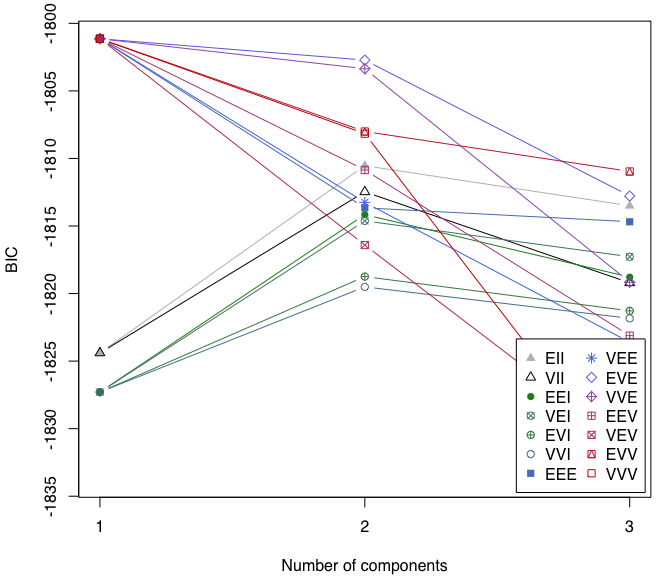``````plot(dens, what = "density")
points(x, cex = 0.3)
abline(h = 0, v = 0, lty = 3)``````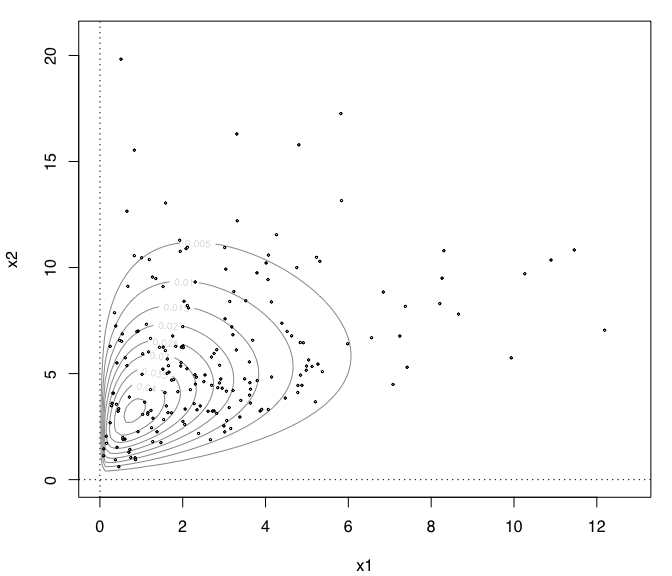``````plot(dens, what = "density", type = "hdr")
abline(h = 0, v = 0, lty = 3)``````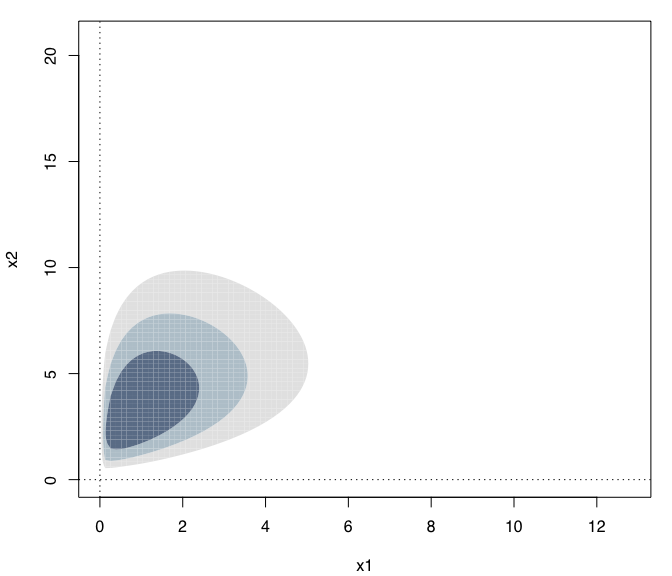``plot(dens, what = "density", type = "persp")``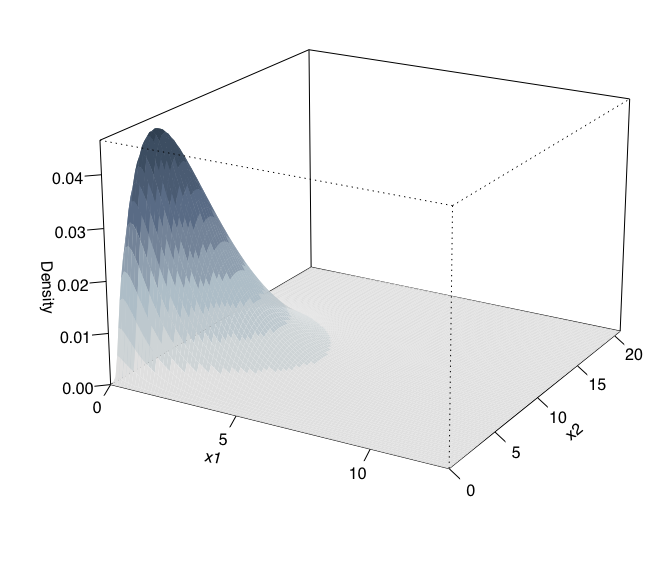## Suicide data

The data consist in the lengths of 86 spells of psychiatric treatment undergone by control patients in a suicide study (Silverman, 1986).

``````data("suicide")
dens <- densityMclustBounded(suicide, lbound = 0)
summary(dens, parameters = TRUE)
## ── Density estimation for bounded data via GMMs ───────────
##
## Boundaries: suicide
##       lower       0
##       upper     Inf
##
## Model E (univariate, equal variance) model with 1 component
## on the transformation scale:
##
##  log-likelihood  n df       BIC       ICL
##       -497.8204 86  3 -1009.004 -1009.004
##
##                               suicide
## Range-power transformation: 0.1929267
##
## Mixing probabilities:
## 1
## 1
##
## Means:
##        1
## 6.700073
##
## Variances:
##        1
## 7.788326
plot(dens, what = "density",
lwd = 2, col = "dodgerblue2",
data = suicide, breaks = 15,
xlab = "Length of psychiatric treatment")
rug(suicide)``````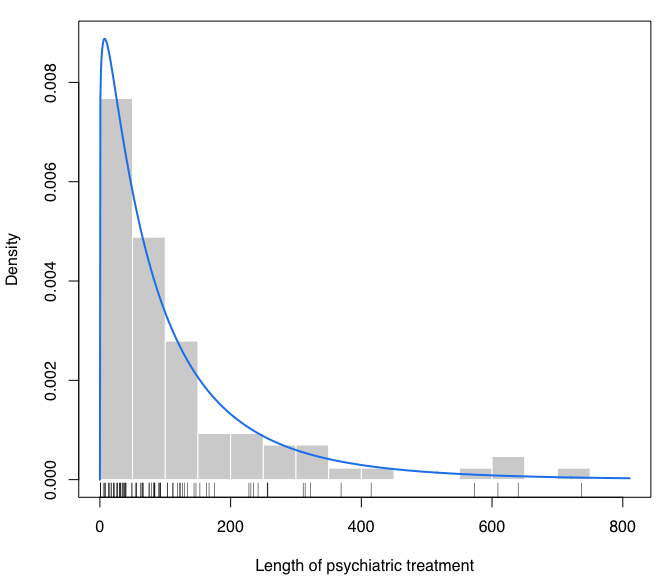## Racial data

This dataset provides the proportion of white student enrollment in 56 school districts in Nassau County (Long Island, New York), for the 1992-1993 school year (Simonoff 1996, Sec. 3.2).

``````data("racial")
x <- racial\$PropWhite
dens <- densityMclustBounded(x, lbound = 0, ubound = 1)
summary(dens, parameters = TRUE)
## ── Density estimation for bounded data via GMMs ───────────
##
## Boundaries: x
##       lower 0
##       upper 1
##
## Model E (univariate, equal variance) model with 1 component
## on the transformation scale:
##
##  log-likelihood  n df      BIC      ICL
##         42.4598 56  3 72.84355 72.84355
##
##                                     x
## Range-power transformation: 0.3869476
##
## Mixing probabilities:
## 1
## 1
##
## Means:
##        1
## 2.795429
##
## Variances:
##        1
## 5.253254
plot(dens, what = "density",
lwd = 2, col = "dodgerblue2",
data = x, breaks = 15,
xlab = "Proportion of white student enrolled in schools")
rug(x)``````